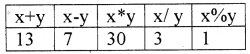# Plus One Computer Science Notes Chapter 6 Data Types and Operators

Students can Download Chapter 6 Data Types and Operators Notes, Plus One Computer Science Notes helps you to revise the complete Kerala State Syllabus and score more marks in your examinations.

## Kerala Plus One Computer Science Notes Chapter 6 Data Types and Operators

Summary
Concepts of data types:
The nature of data is different, data type specifies the nature of data we have to store.

C++ data types:Fundamental data types:
It is also called built in data type. They are int, char, float, double and void
1. int data type:
It is used to store whole numbers without fractional (decimal point) part. It can be either negative or positive. It consumes 4 bytes (32 bits) of memory. i.e. 232 numbers. That is 231 negative numbers and 231 positive numbers (0 is considered as +ve) So a total of 232 numbers. We can store a number in between -231 to + 231-.

2. char data type:
Any symbol from the keyboard, eg: ‘A’, ‘?’, ‘9’ and so on. It consumes one byte( 8 bits) of memory. It is internally treated as integers, i.e. 28 = 256 characters. Each character is having a ASCII code, ‘a’ is having ASCII code 97 and zero is having ASCII code 48.

3. float data type:
It is used to store real numbers i.e. the numbers with decimal point. It uses 4 bytes(32 bits) of memory. eg: 67.89, 89.9 E-15.

4. double data type:
It is used to store very large real numbers. It uses 8 bytes(64 bits) of memory.

5. void data type:
void means nothing. It is used to represent a function returns nothing.

1. User defined Data types: C++ allows programmers to define their own data type. They are Structure(struct), enumeration (enum), union, class, etc.
2. Derived data types: The data types derived from fundamental data types are called Derived data types. They are Arrays, pointers, functions, etcVariables:
The named memory locations are called variable. A variable has three important things

1. variable name: A variable should have a name
2. Memory address: Each and every byte of memory has an address. It is also called location (L) value.
3. Content: The value stored in a variable is called content. lt is also called Read(R) value.

Operators:
An operator is a symbol that performs an operation. The data on which operations are carried out are called operands. Following are the operators
1. lnput(>>) and output(<<) operators are used to perform input and output operation.
eg: cin>>n;
cout<<n;

2. Arithmetic operators:
It is a binary operator. It is used to perform addition(+), subtraction(-), division (/), multiplication(*) and modulus(%- gives the remainder) operations.
eg: If x = 10 and y = 3 thenx/y = 3, because both operands are integer. To get the floating point result one of the operand must be float.

3. Relational operator:
It is also a binary operator. It is used to perform comparison or relational operation between two values and it gives either true(1) or false(O). The operators are <, <=, >, >=, == (equality)and !=(not equal to)
eg: If x = 10 and y = 3 then4. Logical operators:
Here AND(&&) , OR(||) are binary operators and NOT (!) is a unary operator. It is used to combine relational operations and it gives either true(1) orfalse(O). If x = 1 and y = 0 thenBoth operands must be true to get a true value in the case of AND (&&) operation. If x = 1 and y = 0 thenEither one of the operands must be true to get a true value in the case of OR(||) operation. If x = 1 and y = 0 then

 !x !y 0 15. Conditional operator:
It is a ternary operator hence it needs three operands. The operator is”?:”.
Syntax:
expression ? value if true : value if false. First evaluates the expression if it is true the second part will be executed otherwise the third part will be executed.
eg: If x = 10 and y = 3 then x>y ? cout<<x : cout<<y;
Here the output is 10

6. sizeof():
This operator is used to find the size used by each data type. eg: sizeof(int) gives 2.

7. Increment and decrement operator:
These are unary operators.

• Increment operator (++): It is used to increment the value of a variable by one i.e., x++ is equivalent to x = x + 1.
• Decrement operator (- -): It is used to decrement the value of a variable by one i.e., x – – is equivalent to x = x – 1.

8. Assignment operator (=):
lt is used to assign the value of a right side to the left side variable.eg. x = 5; Here the value 5 is assigned to the variable x.

Expressions:
It is composed of operators and operandsArithmetic expression:
It is composed of arithmetic operators and operands. In this expression the operands are integers then it is called Integer expression. If the operands are real numbers then it is called Floating point expression. If the operands are constants then it is called constant expression.

Relational expression:
It is composed of relational operators and operands

Logical expression:
It is composed of logical operators and operands

Statements:
Statements are smallest executable unit of a programming language. Each and every statement must be end with semicolon(;).

Declaration statement:
Each and every variable must be declared before using it. eg: int age;

Assignment statements:
Assignment operator is used to assign the value of RHS to LHS. eg: x = 100

Input statements:
lnput(>>) operator is used to perform input operation. eg: cin>>n;

Output statements:
output(<<) operator is used to perform output operation. eg: cout<<n;